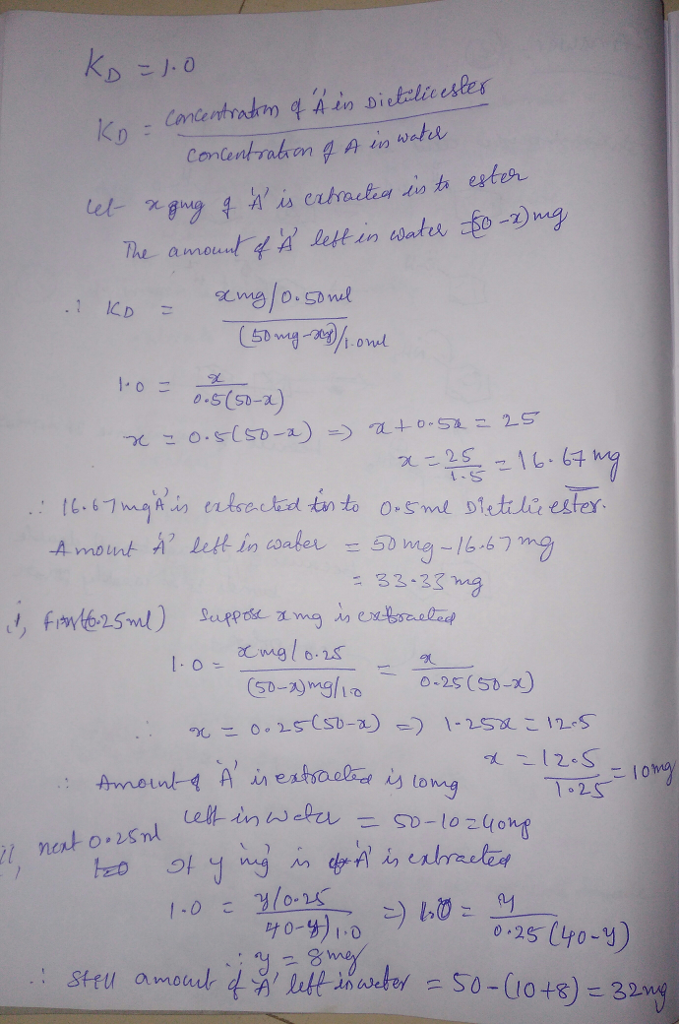# Homework Solution: EXTRACTION EXERCISE. The Kd (coeficient of distribution) between Dietilic eter and Water…

EXTRACTION EXERCISE. The Kd (coeficient of distribution) between Dietilic eter and Water is 1.0 if 50mg of A is dissolve on 1.0ml of water. How much of A is going to be extracted in a portion of 0.50ml of dietilic eter? How much of A is going to remain on the water? Remember Kd=1.0 I have already post this first part , NOW is another question and Im reposting it for this next one only and is How much is going to be extracted with two portions of 0.25ml of dietilic eter? How much of A is going to remain on Water?